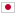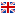Contest Duration: - (local time) (300 minutes) Back to Home
J - AB Sort/Time Limit: 2 sec / Memory Limit: 1024 MB

Score : 100 points

### Problem Statement

You have a string s=s_0s_1...s_{N-1} of length N consisting of A and B. You have to process Q queries. Consider the i-th query ( 1 \leq i \leq Q ). In this query you are given integers l_i and r_i. Then, for each x ( l_i \leq x \leq r_i ), s_x is changed to the other letter, that is, A becomes B and B becomes A.

After each query, you have to calculate f(B + s + A). Here, f(t) is a function which, given a string t, returns a non-negative integer. The value of f(t) is defined as follows:

• Consider the following step.
• Step: For all substrings of t which coincide with BA, replace them with AB. All replacements are done at the same time.
• f(t) is the number of steps you need to perform until no substring of t coincides with BA.

For example, f(ABAB) = 1, f(BBAA) = 3, and f(AAA) = 0.

### Constraints

• 1 \leq N \leq 200000
• |s| = N
• s consists of A and B.
• 1 \leq Q \leq 200000
• 0 \leq l_i \leq r_i \leq N-1
• N,Q,l_i,r_i are integers.

### Input

Input is given from Standard Input in the following format:

N
s
Q
l_1 r_1
l_2 r_2
:
l_Q r_Q


### Output

After each query, print the value of f(B + s + A), one per line.

### Sample Input 1

5
BAABA
2
1 3
0 2


### Sample Output 1

6
6


After the first query, the string s is BBBAA, so print the value of f(BBBBAAA) in the first line. After the second query, the string s is AAAAA, so print the value of f(BAAAAAA) in the second line.

### Sample Input 2

1
A
2
0 0
0 0


### Sample Output 2

2
2